# modwt

Maximal overlap discrete wavelet transform

## Syntax

``w = modwt(x)``
``w = modwt(x,wname)``
``w = modwt(x,Lo,Hi)``
``w = modwt(___,lev)``
``w = modwt(___,'reflection')``
``w = modwt(___,TimeAlign=alignflag)``

## Description

example

````w = modwt(x)` returns the maximal overlap discrete wavelet transform (MODWT) of `x`. `x` can be a real- or complex-valued vector or matrix. If `x` is a matrix, `modwt` operates on the columns of `x`. `modwt` computes the wavelet transform down to level `floor(log2(length(x)))` if `x` is a vector and `floor(log2(size(x,1)))` if `x` is a matrix. By default, `modwt` uses the Daubechies least-asymmetric wavelet with four vanishing moments (`'sym4'`) and periodic boundary handling. ```

example

````w = modwt(x,wname)` uses the orthogonal wavelet, `wname`, for the MODWT. ```

example

````w = modwt(x,Lo,Hi)` uses the scaling filter, `Lo`, and wavelet filter, `Hi`, to compute the MODWT. These filters must satisfy the conditions for an orthogonal wavelet. You cannot specify both `wname` and a filter pair, `Lo` and `Hi`.```

example

``` `w = modwt(___,lev)` computes the MODWT down to the specified level, `lev`, using any of the arguments from previous syntaxes. ```

example

````w = modwt(___,'reflection')` computes the MODWT using reflection boundary handling. Other inputs can be any of the arguments from previous syntaxes. Before computing the wavelet transform, `modwt` extends the signal symmetrically at the terminal end to twice the signal length. The number of wavelet and scaling coefficients that `modwt` returns is equal to twice the length of the input signal. By default, the signal is extended periodically.You must enter the entire character vector `'reflection'`. If you added a wavelet named `'reflection'` using the wavelet manager, you must rename that wavelet prior to using this option. `'reflection'` may be placed in any position in the input argument list after `x`.```

example

````w = modwt(___,TimeAlign=alignflag)` circularly shifts the wavelet coefficients at all levels (scales) and the scaling coefficients to correct for the delay of the scaling and wavelet filters. Other inputs can be any of the arguments from previous syntaxes.```

## Examples

collapse all

Obtain the MODWT of an electrocardiogram (ECG) signal using the default `sym4` wavelet down to the maximum level. The data are taken from Percival & Walden (2000), p.125 (data originally provided by William Constantine and Per Reinhall, University of Washington).

```load wecg; wtecg = modwt(wecg); whos wtecg```
``` Name Size Bytes Class Attributes wtecg 12x2048 196608 double ```

The first eleven rows of `wtecg` are the wavelet coefficients for scales ${2}^{1}$ to ${2}^{11}$. The final row contains the scaling coefficients at scale ${2}^{11}$. Plot the detail (wavelet) coefficients for scale ${2}^{3}$.

```plot(wtecg(3,:)) title('Level 3 Wavelet Coefficients')```Obtain the MODWT of Southern Oscillation Index data with the `db2` wavelet down to the maximum level.

```load soi; wsoi = modwt(soi,'db2');```

Obtain the MODWT of the Deutsche Mark - U.S. Dollar exchange rate data using the Fejér-Korovkin length 8 scaling and wavelet filters.

```load DM_USD [~,~,Lo,Hi] = wfilters("fk8"); wdmf = modwt(DM_USD,Lo,Hi);```

Obtain a second MODWT with the same wavelet, but this time specify the wavelet name.

`wdmn = modwt(DM_USD,"fk8");`

Confirm the decompositions are equal.

`max(abs(wdmf(:)-wdmn(:)))`
```ans = 0 ```

Obtain the MODWT of an ECG signal down to scale ${2}^{4}$, which corresponds to level four. Use the default `sym4` wavelet. The data are taken from Percival & Walden (2000), p.125 (data originally provided by William Constantine and Per Reinhall, University of Washington).

```load wecg; wtecg = modwt(wecg,4); whos wecg wtecg```
``` Name Size Bytes Class Attributes wecg 2048x1 16384 double wtecg 5x2048 81920 double ```

The row size of `wtecg` is L+1, where, in this case, the level (L) is 4. The column size matches the number of input samples.

Obtain the MODWT of an ECG signal using reflection boundary handling. Use the default `sym4` wavelet and obtain the transform down to level 4. The data are taken from Percival & Walden (2000), p.125 (data originally provided by William Constantine and Per Reinhall, University of Washington).

```load wecg; wtecg = modwt(wecg,4,'reflection'); whos wecg wtecg```
``` Name Size Bytes Class Attributes wecg 2048x1 16384 double wtecg 5x4096 163840 double ```

`wtecg` has 4096 columns, which is twice the length of the input signal, `wecg`.

Create a unit impulse signal.

```n = 128; sig = zeros(1,n); sig(n/2) = 1; clf plot(sig) axis tight title("Unit Impulse")```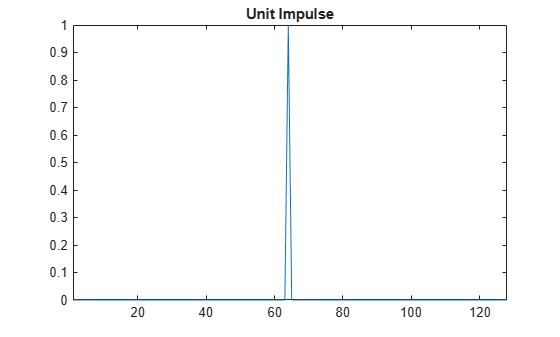Obtain the MODWT of the signal down to level 4 using default `modwt` settings. Obtain a second MODWT of the signal down to level 4 with the coefficients time aligned.

```lev = 4; w = modwt(sig,lev); wTimeAligned = modwt(sig,lev,TimeAlign=true);```

Plot the wavelet and scaling coefficients of the MODWT obtained with default settings. The delays increase with scale because the wavelet and scaling filters used in the MODWT have non-zero phase response.

```for k=1:lev+1 subplot(lev+1,1,k) plot(w(k,:)) axis tight if k==1 title("MODWT Analysis with Delay") end end```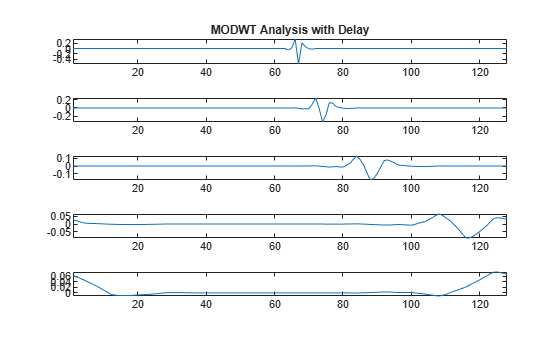Compare with plots of the time-aligned coefficients.

```for k=1:lev+1 subplot(lev+1,1,k) plot(wTimeAligned(k,:)) axis tight if k==1 title("Time-Aligned MODWT Analysis") end end```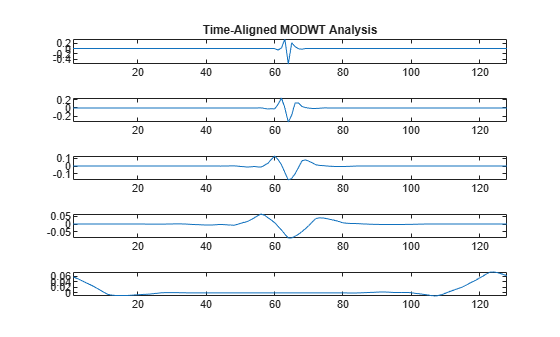Load the 23 channel EEG data `Espiga3` . The channels are arranged column-wise. The data is sampled at 200 Hz.

`load Espiga3`

Compute the maximal overlap discrete wavelet transform down to the maximum level.

`wt = modwt(Espiga3);`

Obtain the squared signal energies and compare them against the squared energies obtained from summing the wavelet coefficients over all levels. Use the log-squared energy due to the disproportionately large energy in one component.

```sigN2 = vecnorm(Espiga3).^2; wtN2 = sum(squeeze(vecnorm(wt,2,2).^2)); bar(1:23,log(sigN2)) hold on scatter(1:23,log(wtN2),'filled','SizeData',100) alpha(0.75) legend('Signal Energy','Energy in Wavelet Coefficients', ... 'Location','NorthWest') xlabel('Channel') ylabel('ln(squared energy)')```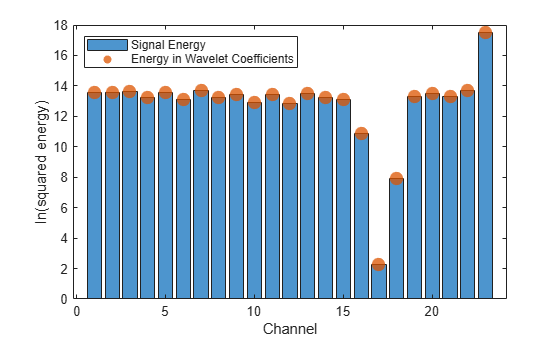This example demonstrates the differences between the MODWT and MODWTMRA. The MODWT partitions a signal's energy across detail coefficients and scaling coefficients. The MODWTMRA projects a signal onto wavelet subspaces and a scaling subspace.

Choose the `sym6` wavelet. Load and plot an electrocardiogram (ECG) signal. The sampling frequency for the ECG signal is 180 hertz. The data are taken from Percival and Walden (2000), p.125 (data originally provided by William Constantine and Per Reinhall, University of Washington).

```load wecg t = (0:numel(wecg)-1)/180; wv = 'sym6'; plot(t,wecg) grid on title(['Signal Length = ',num2str(numel(wecg))]) xlabel('Time (s)') ylabel('Amplitude')```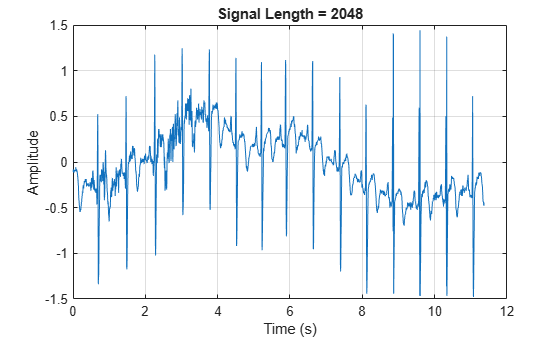Take the MODWT of the signal.

`wtecg = modwt(wecg,wv);`

The input data are samples of a function $f\left(x\right)$ evaluated at $N$ time points. The function can be expressed as a linear combination of the scaling function $\varphi \left(x\right)$ and wavelet $\psi \left(x\right)$at varying scales and translations: $f\left(x\right)=\sum _{k=0}^{N-1}{c}_{k}\phantom{\rule{0.16666666666666666em}{0ex}}{2}^{-{J}_{0}/2}\varphi \left({2}^{-{J}_{0}}\phantom{\rule{0.16666666666666666em}{0ex}}x-k\right)+\sum _{j=1}^{{J}_{0}}{f}_{j}\left(x\right),$ where ${f}_{j}\left(x\right)=\sum _{k=0}^{N-1}{d}_{j,k}\phantom{\rule{0.16666666666666666em}{0ex}}{2}^{-j/2}\phantom{\rule{0.16666666666666666em}{0ex}}\psi \left({2}^{-j}x-k\right)$ and ${J}_{0}$ is the number of levels of wavelet decomposition. The first sum is the coarse scale approximation of the signal, and the ${f}_{j}\left(x\right)$ are the details at successive scales. MODWT returns the $N$ coefficients $\left\{{c}_{k}\right\}$and the $\left({J}_{0}×N\right)$ detail coefficients $\left\{{d}_{j,k}\right\}$ of the expansion. Each row in `wtecg` contains the coefficients at a different scale.

When taking the MODWT of a signal of length $N,$ there are $\text{floor}\left({\mathrm{log}}_{2}\left(N\right)\right)$ levels of decomposition by default. Detail coefficients are produced at each level. Scaling coefficients are returned only for the final level. In this example, $N=2048$, ${J}_{0}=\text{floor}\left(\mathrm{log}2\left(2048\right)\right)=11,$ and the number of rows in `wtecg` is ${J}_{0}+1=11+1=12$.

The MODWT partitions the energy across the various scales and scaling coefficients: ${||X||}^{2}=\sum _{j=1}^{{J}_{0}}{||{W}_{j}||}^{2}+{||{V}_{{J}_{0}}||}^{2},$ where $X$ is the input data, ${W}_{j}$ are the detail coefficients at scale $j$, and ${V}_{{J}_{0}}$ are the final-level scaling coefficients.

Compute the energy at each scale, and evaluate their sum.

```energy_by_scales = sum(wtecg.^2,2); Levels = {'D1';'D2';'D3';'D4';'D5';'D6';... 'D7';'D8';'D9';'D10';'D11';'A11'}; energy_table = table(Levels,energy_by_scales); disp(energy_table)```
``` Levels energy_by_scales _______ ________________ {'D1' } 14.063 {'D2' } 20.612 {'D3' } 37.716 {'D4' } 25.123 {'D5' } 17.437 {'D6' } 8.9852 {'D7' } 1.2906 {'D8' } 4.7278 {'D9' } 12.205 {'D10'} 76.428 {'D11'} 76.268 {'A11'} 3.4192 ```
`energy_total = varfun(@sum,energy_table(:,2))`
```energy_total=table sum_energy_by_scales ____________________ 298.28 ```

Confirm the MODWT is energy-preserving by computing the energy of the signal and comparing it with the sum of the energies over all scales.

```energy_ecg = sum(wecg.^2); max(abs(energy_total.sum_energy_by_scales-energy_ecg))```
```ans = 7.4402e-10 ```

Take the MODWTMRA of the signal.

`mraecg = modwtmra(wtecg,wv);`

MODWTMRA returns the projections of the function $f\left(x\right)$ onto the various wavelet subspaces and final scaling space. That is, MODWTMRA returns $\sum _{k=0}^{N-1}{c}_{k}\phantom{\rule{0.16666666666666666em}{0ex}}{2}^{-{J}_{0}/2}\varphi \left({2}^{-{J}_{0}}\phantom{\rule{0.16666666666666666em}{0ex}}x-k\right)$ and the ${J}_{0}$-many $\left\{{f}_{j}\left(x\right)\right\}$evaluated at $N$ time points. Each row in `mraecg` is a projection of $f\left(x\right)$ onto a different subspace. This means the original signal can be recovered by adding all the projections. This is not true in the case of the MODWT. Adding the coefficients in `wtecg` will not recover the original signal.

Choose a time point, add the projections of $f\left(x\right)$ evaluated at that time point, and compare with the original signal.

```time_point = 1000; abs(sum(mraecg(:,time_point))-wecg(time_point))```
```ans = 3.0846e-13 ```

Confirm that, unlike MODWT, MODWTMRA is not an energy-preserving transform.

```energy_ecg = sum(wecg.^2); energy_mra_scales = sum(mraecg.^2,2); energy_mra = sum(energy_mra_scales); max(abs(energy_mra-energy_ecg))```
```ans = 115.7053 ```

The MODWTMRA is a zero-phase filtering of the signal. Features will be time-aligned. Show this by plotting the original signal and one of its projections. To better illustrate the alignment, zoom in.

```plot(t,wecg,'b') hold on plot(t,mraecg(4,:),'-') hold off grid on xlim([4 8]) legend('Signal','Projection','Location','northwest') xlabel('Time (s)') ylabel('Amplitude')```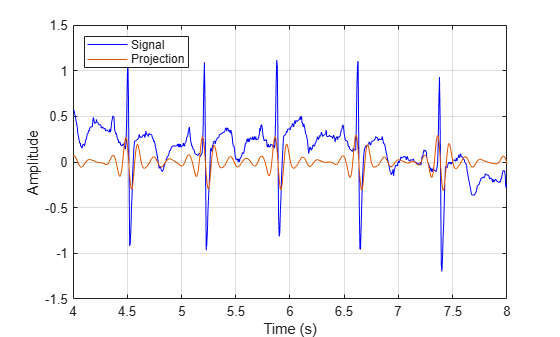Make a similar plot using the MODWT coefficients at the same scale. Features will not be time-aligned. The MODWT is not a zero-phase filtering of the input.

```plot(t,wecg,'b') hold on plot(t,wtecg(4,:),'-') hold off grid on xlim([4 8]) legend('Signal','Coefficients','Location','northwest') xlabel('Time (s)') ylabel('Amplitude')```## Input Arguments

collapse all

Input signal, specified as a vector or matrix. If `x` is a vector, `x` must have at least two elements. If `x` is a matrix, the row dimension of `x` must be at least 2.

Data Types: `single` | `double`
Complex Number Support: Yes

Wavelet, specified as a character vector or string scalar. The wavelet must be orthogonal. Orthogonal wavelets are designated as type 1 wavelets in the wavelet manager, `wavemngr`.

Valid built-in orthogonal wavelet families are: Best-localized Daubechies (`"bl"`), Beylkin (`"beyl"`), Coiflets (`"coif"`), Daubechies (`"db"`), Fejér-Korovkin (`"fk"`), Haar (`"haar"`), Han linear-phase moments (`"han"`), Morris minimum-bandwidth (`"mb"`), Symlets (`"sym"`), and Vaidyanathan (`"vaid"`).

For a list of wavelets in each family, see `wfilters`. You can also use `waveinfo` with the wavelet family short name. For example, `waveinfo("db")`. Use `wavemngr("type",wn)` to determine if the wavelet wn is orthogonal (returns 1). For example, `wavemngr("type","db6")` returns 1.

Filters, specified as a pair of even-length real-valued vectors. `Lo` is the scaling filter, and `Hi` is the wavelet filter. The filters must satisfy the conditions for an orthogonal wavelet. The lengths of `Lo` and `Hi` must be equal. See `wfilters` for additional information. You cannot specify both `wname` and a filter pair `Lo,Hi`.

Note

To agree with the usual convention in the implementation of `modwt` in numerical packages, the roles of the analysis and synthesis filters returned by `wfilters` are reversed in `modwt`. See MODWT Using Scaling and Wavelet Filters.

Data Types: `single` | `double`

Transform level, specified as a positive integer less than or equal to `floor(log2(N))`, where ```N = length(x)``` if `x` is a vector, or ```N = size(x,1)``` if `x` is a matrix. If unspecified, `lev` defaults to `floor(log2(N))`.

Time align coefficients logical which determines whether the MODWT circularly shifts the wavelet coefficients at all levels (scales) and the scaling coefficients to correct for the delay of the scaling and wavelet filters, specified as a numeric or logical `1` (`true`) or `0` (`false`). Coefficients are shifted using the "center of energy" method of Hess-Nielsen and Wickerhauser . Shifting the coefficients is useful if you want to time align features in the signal with the wavelet coefficients.

If you want to reconstruct the signal with the `imodwt` function, or obtain a multiresolution analysis using the `modwtmra` function, do not shift the coefficients. In those cases, the time alignment is performed in obtaining the inverse or multiresolution analysis.

Data Types: `logical`

## Output Arguments

collapse all

MODWT transform of `x`. `w` contains the wavelet coefficients and final-level scaling coefficients of `x`. If `x` is a vector, `w` is a `lev`+1-by-N matrix. If `x` is a matrix, `w` is a `lev`+1-by-N-by-NC array, where NC is the number of columns in `x`. N is equal to the input signal length unless you specify `'reflection'` boundary handling, in which case N is twice the length of the input signal. The kth row of the array, `w`, contains the wavelet coefficients for scale 2k (wavelet scale 2(k-1)). The final, (`lev`+1)th, row contains the scaling coefficients for scale 2`lev`.

## Algorithms

The standard algorithm for the MODWT implements the circular convolution directly in the time domain. This implementation of the MODWT performs the circular convolution in the Fourier domain. The wavelet and scaling filter coefficients at level j are computed by taking the inverse discrete Fourier transform (DFT) of a product of DFTs. The DFTs in the product are the signal’s DFT and the DFT of the jth level wavelet or scaling filter.

Let Hk and Gk denote the length N DFTs of the MODWT wavelet and scaling filters, respectively. Let j denote the level and N denote the sample size.

The jth level wavelet filter is defined by

`$\frac{1}{N}\sum _{k=0}^{N-1}{H}_{j,k}{e}^{i2\pi nk/N}$`

where

`${H}_{j,k}={H}_{{2}^{j-1}k\text{mod}N}\prod _{m=0}^{j-2}{G}_{{2}^{m}k\text{mod}N}$`

The jth level scaling filter is

`$\frac{1}{N}\sum _{k=0}^{N-1}{G}_{j,k}{e}^{i2\pi nk/N}$`

where

`${G}_{j,k}=\prod _{m=0}^{j-1}{G}_{{2}^{m}k\text{mod}N}$`

 Percival, Donald B., and Andrew T. Walden. Wavelet Methods for Time Series Analysis. Cambridge Series in Statistical and Probabilistic Mathematics. Cambridge ; New York: Cambridge University Press, 2000.

 Percival, Donald B., and Harold O. Mofjeld. “Analysis of Subtidal Coastal Sea Level Fluctuations Using Wavelets.” Journal of the American Statistical Association 92, no. 439 (September 1997): 868–80. https://doi.org/10.1080/01621459.1997.10474042.

 Mesa, Hector. “Adapted Wavelets for Pattern Detection.” In Progress in Pattern Recognition, Image Analysis and Applications, edited by Alberto Sanfeliu and Manuel Lazo Cortés, 3773:933–44. Berlin, Heidelberg: Springer Berlin Heidelberg, 2005. https://doi.org/10.1007/11578079_96.

 Hess-Nielsen, N., and M.V. Wickerhauser. “Wavelets and Time-Frequency Analysis.” Proceedings of the IEEE 84, no. 4 (April 1996): 523–40. https://doi.org/10.1109/5.488698.